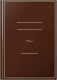Books Books## Adams's New Arithmetic: Arithmetic, in which the Principles of Operating by ...

Daniel Adams - Arithmetic - 1899 - 264 pages## Adams's New Arithmetic: Arithmetic, in which the Principles of Operating by ...

Daniel Adams - Arithmetic - 1844 - 261 pages## Adams's New Arithmetic: Arithmetic, in which the Principles of Operating by ...

Daniel Adams - Arithmetic - 1843 - 261 pages## Adams's New Arithmetic: Arithmetic, in which the Principles of Operating by ...

Daniel Adams - Arithmetic - 1842 - 264 pages## Adams's New Arithmetic: Arithmetic, in which the Principles of Operating by ...

Daniel Adams - Arithmetic - 1839 - 264 pages## Adams's New Arithmetic: Arithmetic, in which the Principles of Operating by ...

Daniel Adams - Arithmetic - 1837 - 264 pages## Adams's New Arithmetic: Arithmetic, in which the Principles of Operating by ...

Daniel Adams - Arithmetic - 1836 - 264 pages## Adams's New Arithmetic: Arithmetic, in which the Principles of Operating by ...

Daniel Adams - Arithmetic - 1835 - 264 pages